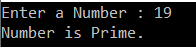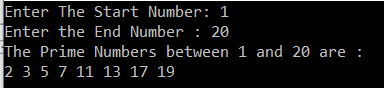# Prime Numbers in C#

## Prime Numbers in C# with Examples

In this article, I am going to discuss the Prime Numbers in C# with Examples. Please read our previous article where we discussed the Fibonacci Series Program with some examples. C# prime number example program is one of the most frequently asked written exam interview questions in C# Interview. As part of this article, we are going to discuss the following pointers.

1. What is a Prime Number?
2. How to check if a given number is prime or not in C#?
3. How to Print the Prime Numbers between a range of numbers in C#?
##### What is a Prime Number?

A Prime Number is a number that should be greater than 1 and it only is divided by 1 and itself. In other words, we can say that the prime numbers can’t be divided by other numbers than itself and 1. For example, 2, 3, 5, 7, 11, 13, 17, 19, 23…., are the prime numbers.

##### How to check if a given number is prime or not in C#?

The following example takes one input from the console and then checks whether that number is a prime number or not.

```using System;
namespace LogicalPrograms
{
public class Program
{
static void Main(string[] args)
{
Console.Write("Enter a Number : ");
bool IsPrime = true;

for (int i = 2; i < number/2; i++)
{
if (number % i == 0)
{
IsPrime = false;
break;
}
}

if (IsPrime)
{
Console.Write("Number is Prime.");
}
else
{
Console.Write("Number is not Prime.");
}
}
}
}
```

Output:##### How to display Prints the Prime Numbers between a range of numbers in C#?

In the following example, we will take two numbers from the console and then print the prime numbers present between those two numbers.

```using System;
namespace LogicalPrograms
{
public class Program
{
static void Main(string[] args)
{
Console.Write("Enter The Start Number: ");
Console.Write("Enter the End Number : ");
Console.WriteLine(\$"The Prime Numbers between {startNumber} and {endNumber} are : ");

for (int i = startNumber; i <= endNumber; i++)
{
int counter = 0;

for (int j = 2; j <= i / 2; j++)
{
if (i % j == 0)
{
counter++;
break;
}
}

if (counter == 0 && i != 1)
{
Console.Write("{0} ", i);
}
}
}
}
}
```

Output:That’s it for today. Here, in this article, I try to explain what is a prime number and how to check whether a number is prime or not using a console application. In the end, we also discussed how to print the prime numbers between a range. In the next article, I am going to discuss how to check whether a number or string is Palindrome or not in C#. I hope you enjoy this Prime Number Program in C# with Examples article.

### 1 thought on “Prime Numbers in C#”

1.In case of 4, your first code outputs it as a prime number, which is incorrect.
for loop condition limit should be i <= number/2